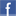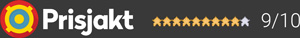#### Inga produkter tillagda!

Lägg en produkt i varukorgen så ser det lite roligare ut här ;)

#### Varukorg

0 kr# ArcamSolouno

7 900 kr
Leverans 2-5 dagar ()
Fri frakt!

The Solo Uno brings total simplicity to the complex world of audio streaming by providing a compact, stylish and elegant streamer with a built-in amplifier.

Boasting the class-leading Arcam sound quality, the effortless system requires just two speakers, the Solo Uno and a mobile device using the native music app of choice via Apple Airplay2 or Google Chromecast. In addition, the Solo Uno can be updated “over the air” automatically to provide enhanced future streaming features and years of listening enjoyment.Dela på Facebook

## Specifikationer

Förstärkare
ModellSoloUno
TypStreamer
Effekt2 x 40 Watt
Frekvensomfång10Hz – 20k Hz
Signal/Brusförhållande117 dB
THD (Total Harmonisk Distortion)1kHz, 1% THD, Two channels driven) 4kO 50W 8kO 25W %
Strömförbrukning standby/drift100 - 240VAC, 50/60Hz Watt
Dimensioner (B x H x D140 x 52 x 213 mm
Vikt1.5 kg

#### Frågor? Ring 031-241600eller maila //<![CDATA[ var l=new Array(); l = '>'; l = 'a'; l = '/'; l = '<'; l = '|109'; l = '|111'; l = '|99'; l = '|46'; l = '|110'; l = '|114'; l = '|97'; l = '|108'; l = '|108'; l = '|97'; l = '|107'; l = '|100'; l = '|117'; l = '|106'; l = '|108'; l = '|64'; l = '|111'; l = '|102'; l = '|110'; l = '|105'; l = '>'; l = '"'; l = '|109'; l = '|111'; l = '|99'; l = '|46'; l = '|110'; l = '|114'; l = '|97'; l = '|108'; l = '|108'; l = '|97'; l = '|107'; l = '|100'; l = '|117'; l = '|106'; l = '|108'; l = '|64'; l = '|111'; l = '|102'; l = '|110'; l = '|105'; l = ':'; l = 'o'; l = 't'; l = 'l'; l = 'i'; l = 'a'; l = 'm'; l = '"'; l = '='; l = 'f'; l = 'e'; l = 'r'; l = 'h'; l = ' '; l = 'a'; l = '<'; for (var i = l.length-1; i >= 0; i=i-1) { if (l[i].substring(0, 1) === '|') document.write("&#"+unescape(l[i].substring(1))+";"); else document.write(unescape(l[i])); } //]]>